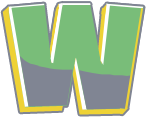Wordle Unlimited
Wordle Numbers and Math: Put your math skills to use. The game uses only numbers and signs addition (+), subtraction (-), multiplication (*), division (/), and equal to (=).# Numbers and Math

Wordle Numbers and Math: Put your math skills to use. The game uses only numbers and signs addition (+), subtraction (-), multiplication (*), division (/), and equal to (=).